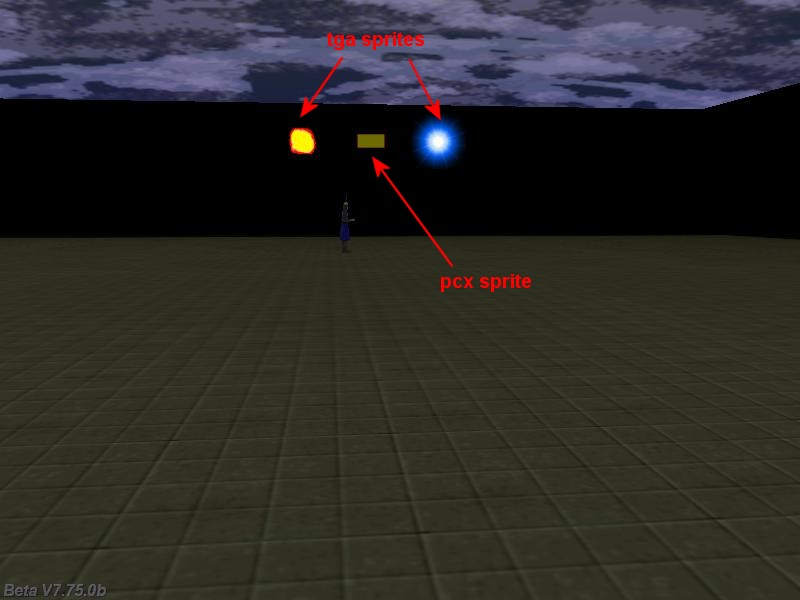New engine features
 New particle system   A new particle system was implemented; the particles are now round instead of square. The BEAM effect is a lot faster and GameStudio Professional Edition uses instancing now, allowing the creation of 10 times more particles without lowering the frame rate.   function vec_randomize (VECTOR* vec, var range) {        vec_set(vec,vector(random(1)-0.5, random(1)-0.5, random(1)-0.5));        vec_normalize(vec, random(range)); }   function particle_fade(PARTICLE *p) {        p.alpha -= 1 * time_step;        if (p.alpha <= 0) p.lifespan = 0; }   function particle_fountain(PARTICLE* p) {        VECTOR vTemp;        vec_randomize(vTemp, 5);        vec_add(p.vel_x, vTemp);        vec_set(p.blue, vector(random(155), random(155), random(255)));        set(p, MOVE | BRIGHT | TRANSLUCENT | BEAM);        p.alpha = 100;        p.size = 2;        p.gravity = 0.3;        p.event = particle_fade; }   action particle_generator() // attach this action to a small model {        set (my, INVISIBLE | PASSABLE);        while(1)        {                effect(particle_fountain, 100, my.x, vector(0, 0, 7)); // generate 100 particles / frame                wait(1);        } }video_alpha   Gives the the transparency factor of the engine window. Can be used to fade the engine window in and out.   function quit_game() {        while (video_alpha > 0)        {                video_alpha -= 1 * time_step; // 1 = fading speed                wait (1);        }        sys_exit(NULL); // shut down the engine }   function quit_startup() {        on_f10 = quit_game; // press the F10 key to shut down the game }     ent_for_file (file_name)   Returns a pointer to the entity with the given file name.   TEXT* sprites_txt = // create a text that can store up to 100 strings {        strings = 100; }   function brighter_startup() // increases the ambient of all the tga files from the game folder {        var i = 0;        while(i < 100) // first of all, let's reset the text strings        {                str_cpy((sprites_txt.pstring)[i], "");                i++;        }        i = 0;        txt_for_dir(sprites_txt, "*.tga"); // now load all the available tga sprite names in the strings        while (str_cmpi((sprites_txt.pstring)[i], "") == 0) // run until all the tga sprites have been processed        {                you = ent_for_file((sprites_txt.pstring)[i]);                if (you) // got a tga file here?                {                        you.ambient = 100; // then increase its ambient to 100!                }                i ++;        } }